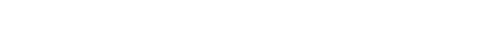glNormal.3glOpenGL man pages

```

```

## Name

```  glNormal3b, glNormal3d, glNormal3f, glNormal3i, glNormal3s, glNormal3bv,
glNormal3dv, glNormal3fv, glNormal3iv, glNormal3sv - set the current normal
vector

```

## C Specification

```  void glNormal3b( GLbyte nx,
GLbyte ny,
GLbyte nz )
void glNormal3d( GLdouble nx,
GLdouble ny,
GLdouble nz )
void glNormal3f( GLfloat nx,
GLfloat ny,
GLfloat nz )
void glNormal3i( GLint nx,
GLint ny,
GLint nz )
void glNormal3s( GLshort nx,
GLshort ny,
GLshort nz )

```

## Parameters

```
nx, ny, nz
Specify the	x, y, and z coordinates	of the new current normal.
The	initial	value of the current normal is (0,0,1).

```

## C Specification

```  void glNormal3bv( const GLbyte *v )
void glNormal3dv( const GLdouble *v )
void glNormal3fv( const GLfloat *v )
void glNormal3iv( const GLint	*v )
void glNormal3sv( const GLshort *v )

```

## Parameters

```
v    Specifies a pointer to an array of three	elements: the x, y, and	z
coordinates of the new current normal.

```

## Description

```  The current normal is	set to the given coordinates whenever glNormal is
issued.  Byte, short,	or integer arguments are converted to floating-point
format with a	linear mapping that maps the most positive representable
integer value	to 1.0,	and the	most negative representable integer value to
-1.0.

Normals specified with glNormal need not have	unit length.  If
normalization	is enabled, then normals specified with	glNormal are
normalized after transformation.  Normalization is controlled	using
glEnable and glDisable with the argument GL_NORMALIZE.  By default,
normalization	is disabled.

```

## Notes

```  The current normal can be updated at any time.  In particular, glNormal can
be called between a call to glBegin and the corresponding call to glEnd.

```

## Associated Gets

```  glGet	with argument GL_CURRENT_NORMAL
glIsEnable with argument GL_NORMALIZE

```

```  glBegin, glColor, glIndex, glTexCoord, glVertex